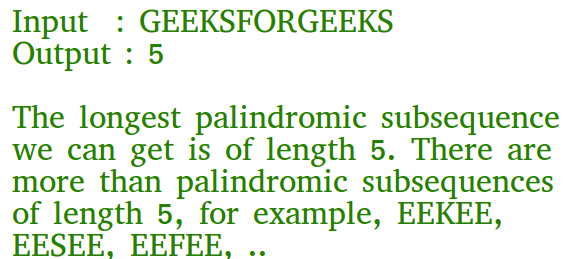# C# Program for Longest Palindromic Subsequence | DP-12

• Last Updated : 13 Aug, 2021

Given a sequence, find the length of the longest palindromic subsequence in it.As another example, if the given sequence is “BBABCBCAB”, then the output should be 7 as “BABCBAB” is the longest palindromic subsequence in it. “BBBBB” and “BBCBB” are also palindromic subsequences of the given sequence, but not the longest ones.

1) Optimal Substructure:
Let X[0..n-1] be the input sequence of length n and L(0, n-1) be the length of the longest palindromic subsequence of X[0..n-1].
If last and first characters of X are same, then L(0, n-1) = L(1, n-2) + 2.
Else L(0, n-1) = MAX (L(1, n-1), L(0, n-2)).

Following is a general recursive solution with all cases handled.

## C#

 `// C# program of above approach` `using` `System;`   `public` `class` `GFG {`   `    ``// A utility function to get max of two integers` `    ``static` `int` `max(``int` `x, ``int` `y)` `    ``{` `        ``return` `(x > y) ? x : y;` `    ``}` `    ``// Returns the length of the longest palindromic subsequence in seq`   `    ``static` `int` `lps(``char``[] seq, ``int` `i, ``int` `j)` `    ``{` `        ``// Base Case 1: If there is only 1 character` `        ``if` `(i == j) {` `            ``return` `1;` `        ``}`   `        ``// Base Case 2: If there are only 2 characters and both are same` `        ``if` `(seq[i] == seq[j] && i + 1 == j) {` `            ``return` `2;` `        ``}`   `        ``// If the first and last characters match` `        ``if` `(seq[i] == seq[j]) {` `            ``return` `lps(seq, i + 1, j - 1) + 2;` `        ``}`   `        ``// If the first and last characters do not match` `        ``return` `max(lps(seq, i, j - 1), lps(seq, i + 1, j));` `    ``}`   `    ``/* Driver program to test above function */` `    ``public` `static` `void` `Main()` `    ``{` `        ``String seq = ``"GEEKSFORGEEKS"``;` `        ``int` `n = seq.Length;` `        ``Console.Write(``"The length of the LPS is "` `+ lps(seq.ToCharArray(), 0, n - 1));` `    ``}` `}`   `// This code is contributed by Rajput-Ji`

Output:

`The length of the LPS is 5`

Dynamic Programming Solution

## C#

 `// A Dynamic Programming based C# Program` `// for the Egg Dropping Puzzle` `using` `System;`   `class` `GFG {`   `    ``// A utility function to get max of` `    ``// two integers` `    ``static` `int` `max(``int` `x, ``int` `y)` `    ``{` `        ``return` `(x > y) ? x : y;` `    ``}`   `    ``// Returns the length of the longest` `    ``// palindromic subsequence in seq` `    ``static` `int` `lps(``string` `seq)` `    ``{` `        ``int` `n = seq.Length;` `        ``int` `i, j, cl;`   `        ``// Create a table to store results` `        ``// of subproblems` `        ``int``[, ] L = ``new` `int``[n, n];`   `        ``// Strings of length 1 are` `        ``// palindrome of length 1` `        ``for` `(i = 0; i < n; i++)` `            ``L[i, i] = 1;`   `        ``// Build the table. Note that the` `        ``// lower diagonal values of table` `        ``// are useless and not filled in` `        ``// the process. The values are` `        ``// filled in a manner similar to` `        ``// Matrix Chain Multiplication DP` `        ``// solution (See` `        ``// https:// www.geeksforgeeks.org/matrix-chain-multiplication-dp-8/` `        ``// cl is length of substring` `        ``for` `(cl = 2; cl <= n; cl++) {` `            ``for` `(i = 0; i < n - cl + 1; i++) {` `                ``j = i + cl - 1;`   `                ``if` `(seq[i] == seq[j] && cl == 2)` `                    ``L[i, j] = 2;` `                ``else` `if` `(seq[i] == seq[j])` `                    ``L[i, j] = L[i + 1, j - 1] + 2;` `                ``else` `                    ``L[i, j] = max(L[i, j - 1], L[i + 1, j]);` `            ``}` `        ``}`   `        ``return` `L[0, n - 1];` `    ``}`   `    ``/* Driver program to test above ` `    ``functions */` `    ``public` `static` `void` `Main()` `    ``{` `        ``string` `seq = ``"GEEKS FOR GEEKS"``;` `        ``int` `n = seq.Length;` `        ``Console.Write(``"The length of the "` `                      ``+ ``"lps is "` `+ lps(seq));` `    ``}` `}`   `// This code is contributed by nitin mittal.`

Output

`The length of the lps is 7`

Please refer complete article on Longest Palindromic Subsequence | DP-12 for more details!

My Personal Notes arrow_drop_up
Recommended Articles
Page :